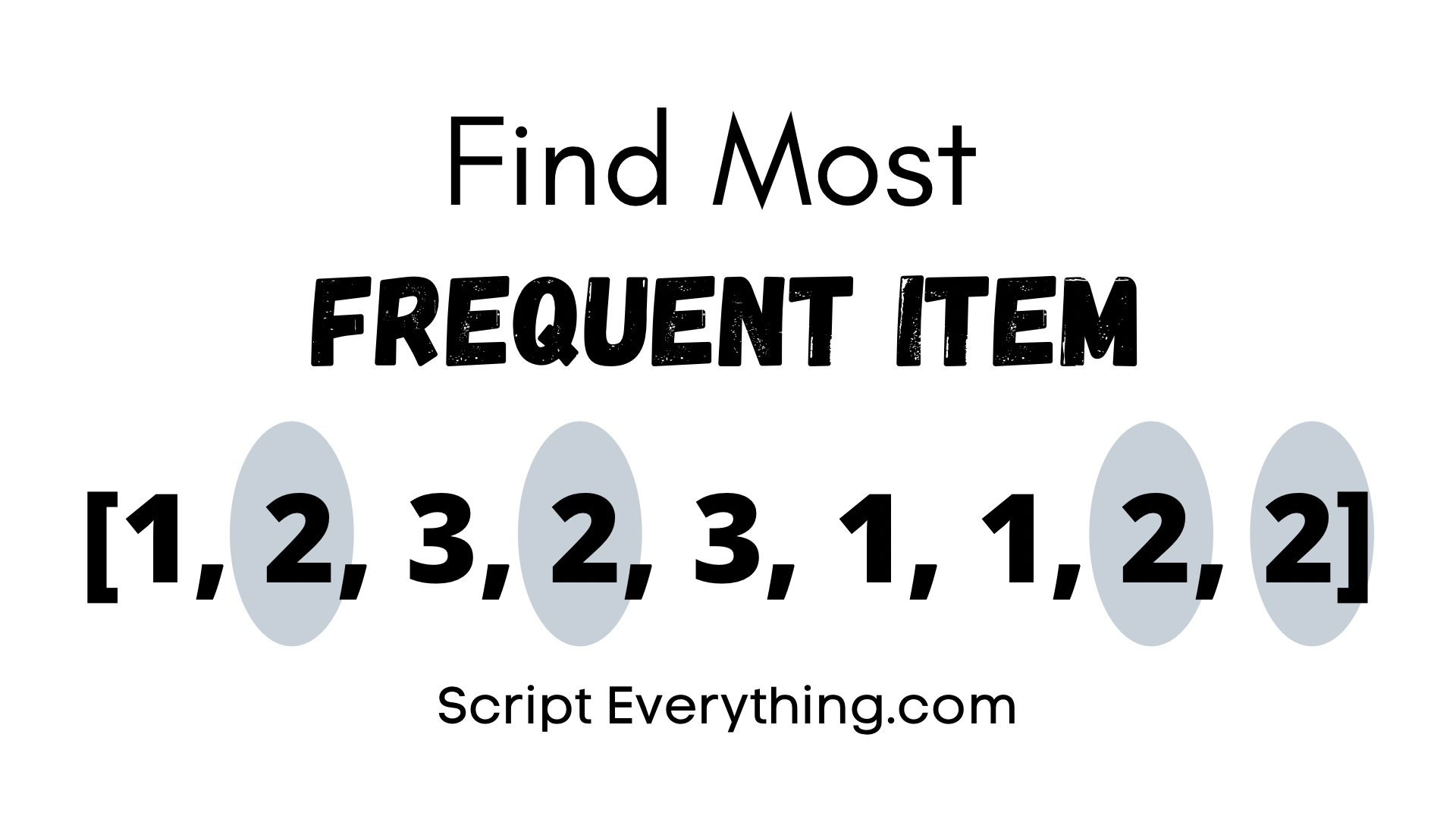# How To Find Most Frequent Element In List In Python (Code Examples, No Import Statements)How do you find the most common element in a list using Python without importing any special libraries?

To find the most frequent elements in a list iterate through the list and store the frequency of each element into a dictionary with the key representing the element and the value representing the frequency. Finally, sort the dictionary by value in descending order to see the results with the highest frequency to the lowest.

Here is a demonstration of how to do this:

``````>>> my_list = ['a', 'b', 'c', 'c']
>>> my_dict = {}
>>> for x in my_list:
...     if x in my_list:
...         my_dict[x] += 1
...     else:
...         my_dict[x] = 1
...
>>> my_dict
{'a': 1, 'b': 1, 'c': 2}``````

From the above example, I initialise a list with some string values, deliberately adding an extra element that already exists in the list. Next, I create an empty dictionary. In the final steps, I create a for-loop that iterates through the list with a simple condition: if the element already exists in the dictionary then increment its value by 1, if it doesn’t exist then create the value in the dictionary and assign it the value of 1.

At the end of the process, I check the value of the dictionary and as you can see it produces the result. The only other step needed was to sort by dictionary value .

To sort the dictionary by value you can use a dictionary comprehension as follows:

``````>>> sorted_dict = {key: value for (key, value) in sorted(my_dict.items(), key=lambda x: x, reverse=True)}
>>> sorted_dict
{'c': 2, 'a': 1, 'b': 1}``````

From this sorting step you can then report the most frequently occurring item in a list by obtaining the first key in the newly created dictionary.

You could do this in one line, and would look like the following:

``````>>> list(sorted_dict)
'c'``````

But what if there were multiple top results, how would you want to display the results if there was more than one result that contained the most common elements in the list?

## Get Most Common Elements From List

If your list could contain more than one result for being the most common element in the list then you would modify your code a little to accommodate.

Here’s an example starting all over again, but this time with more than one result being the correct answer:

``````>>> my_list = ['a', 'b', 'c', 'c', 'b']
>>> my_dict = {}
>>> for x in my_list:
...     if x in my_dict:
...         my_dict[x] += 1
...     else:
...         my_dict[x] = 1
...
>>> sorted_dict = {key: value for (key, value) in sorted(my_dict.items(), key=lambda x: x, reverse=True)}
>>> sorted_dict
{'b': 2, 'c': 2, 'a': 1}``````

As you can see from the example above we have a dictionary with multiple same frequencies. To return the elements with the same top frequency write the following code next:

``````>>> sorted_list = list(sorted_dict)
>>> [x for x in sorted_list if sorted_dict[x] == sorted_dict[sorted_list]]
['b', 'c']``````

The result as seen from the output above shows that only the elements from the list that occur the most frequently are reported.

To report alongside the result what the frequency is make the following amendment to the list comprehension line:

``````>>> [x for x in sorted_list if sorted_dict[x] == sorted_dict[sorted_list]], sorted_dict[sorted_list]
(['b', 'c'], 2)``````

The result being a tuple showing the elements with the most common frequency and then the frequency itself.

## Summary

To find the most frequent element used in a list create a dictionary and iterate through each element reporting its result to the dictionary where the dictionary’s keys represent the elements and the value of each key is the frequency counter.

Then to report the element with the highest frequency simply sort the dictionary by its value in descending order, convert it to a list, and then return the first element from that list.

If it is required to report all elements that have the highest frequency then use a list comprehension on the list from the sorted dictionary to fetch all elements that contain the same frequency.

Author of scripteverything.com, Ryan has been dabbling in code since the late '90s when he cut his teeth by exploring VBA in Excel when trying to do something more. Having his eyes opened with the potential of automating repetitive tasks, he expanded to Python and then moved over to scripting languages such as HTML, CSS, Javascript and PHP. When he is not behind a screen, Ryan enjoys a good bush walk with the family during the cooler months, and going with them to the beach during the warmer months.